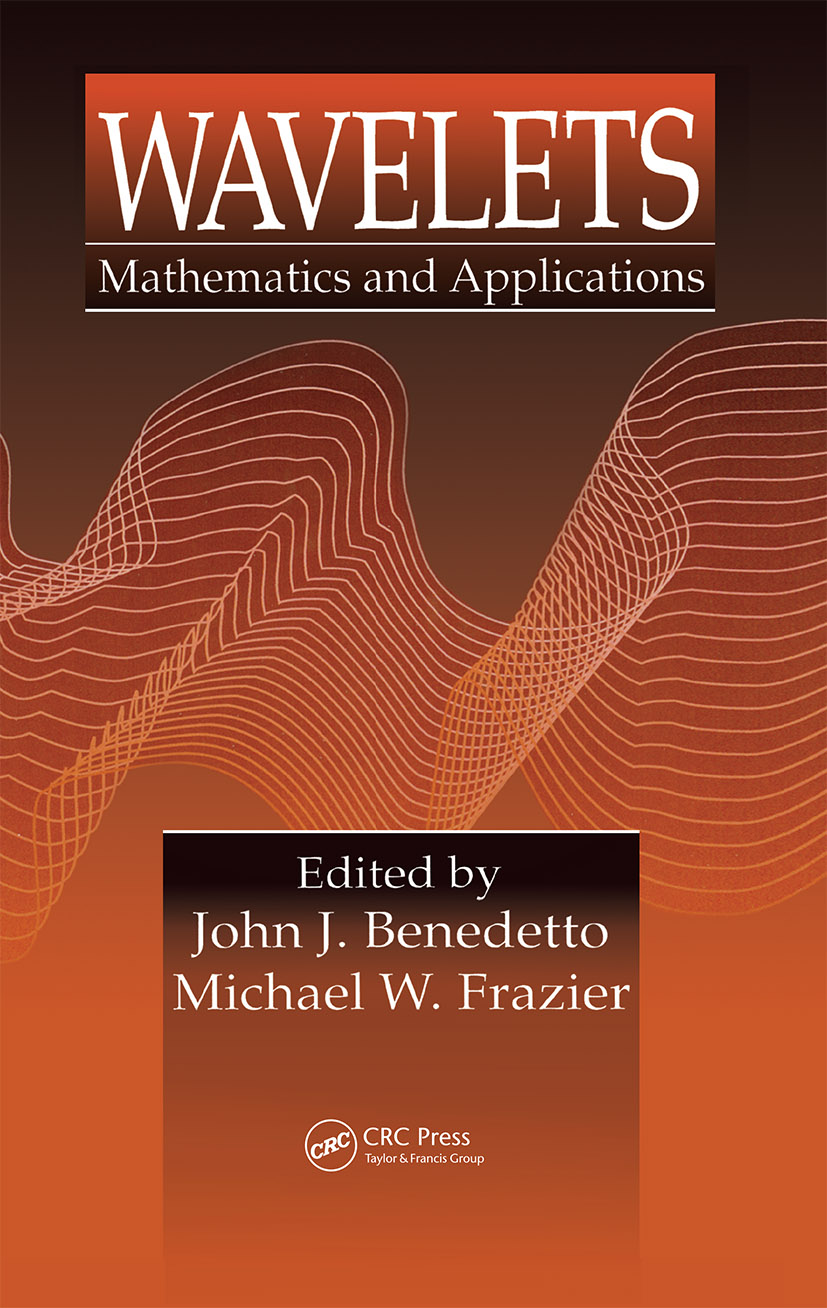Wavelets

Mathematics and Applications, 1st Edition

CRC Press

592 pages

Purchasing Options:\$ = USD
Hardback: 9780849382710
pub: 1993-09-27
SAVE ~\$35.00
\$175.00
\$140.00
x

FREE Standard Shipping!

Description

Wavelets is a carefully organized and edited collection of extended survey papers addressing key topics in the mathematical foundations and applications of wavelet theory. The first part of the book is devoted to the fundamentals of wavelet analysis. The construction of wavelet bases and the fast computation of the wavelet transform in both continuous and discrete settings is covered. The theory of frames, dilation equations, and local Fourier bases are also presented.

The second part of the book discusses applications in signal analysis, while the third part covers operator analysis and partial differential equations. Each chapter in these sections provides an up-to-date introduction to such topics as sampling theory, probability and statistics, compression, numerical analysis, turbulence, operator theory, and harmonic analysis.

The book is ideal for a general scientific and engineering audience, yet it is mathematically precise. It will be an especially useful reference for harmonic analysts, partial differential equation researchers, signal processing engineers, numerical analysts, fluids researchers, and applied mathematicians.

Reviews

"Wavelets is a presentation of the highest quality, of the state of the art in wavelet theory and some of its applications to signal processing and numerical analysis. It is generally easy to read and some of the chapters can be used as introductions to certain aspects of the subject."

-Physics Today, November 1994

Core Material

Construction of Orthonormal Wavelets, R.S. Strichartz

An Introduction to the Orthonormal Wavelet Transform on Discrete Sets, M. Frazier and A. Kumar

Gabor Frames for L2 and Related Spaces, J.J. Benedetto and D.F. Walnut

Dilation Equations and the Smoothness of Compactly Supported Wavelets, C. Heil and D. Colella

Remarks on the Local Fourier Bases, P. Auscher

Wavelets and Signal Processing

The Sampling Theorem, Phi-Transform, and Shannon Wavelets for R, Z, T, and ZN, M. Frazier and R. Torres

Frame Decompositions, Sampling, and Uncertainty Principle Inequalities, J.J. Benedetto

Theory and Practice of Irregular Sampling, H.G. Feichtinger and K. Gröchenig

Wavelets, Probability, and Statistics: Some Bridges, C. Houdré

Wavelets and Adapted Waveform Analysis, R.R. Coifman and V. Wickerhauser

Near Optimal Compression of Orthonormal Wavelet Expansions, B. Jawerth, C.-C. Hsiao, B. Lucier, and X. Yu

Wavelets and Partial Differential Operators

On Wavelet-Based Algorithms for Solving Differential Equations, G. Beylkin

Wavelets and Nonlinear Analysis, S. Jaffard

Scale Decomposition in Burgers' Equation, F. Heurtaux, F. Planchon, and V. Wickerhauser

The Cauchy Singular Integral Operator and Clifford Wavelets, L. Andersson, B. Jawerth, and M. Mitrea

The Use of Decomposition Theorems in the Study of Operators, R. Rochberg